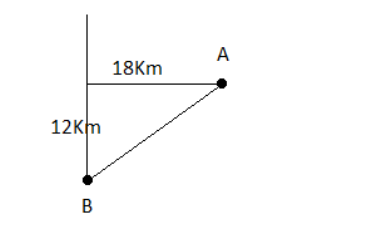# A town B is 12 km south and 18 km west of a town A.`
Question:

A town B is 12 km south and 18 km west of a town A. Show that the bearing of $B$ from $A$ is $S 56^{\circ} 20^{\prime} W .$ Also, find the distance of $B$ from $A .$

Solution:Distance from $A$ to $B$ is $=\sqrt{12^{2}+18^{2}}=\sqrt{468}=21.63 \mathrm{Km}$

Let, bearing from $A$ to $B$ is $\theta$.

So, $\tan \theta=\frac{18}{12}=\frac{3}{2}$

$\theta=\tan ^{-1}\left(\frac{3}{2}\right)=56.31^{\circ}=56^{\circ} 20^{\prime}$